Ch 12. Infinite Sequences and Series Multimedia Engineering Math Sequencesand Series Integral andComparison Alt. Series &Abs. Conv. PowerSeries Taylor, Mac. &Binomial
 Chapter 1. Limits 2. Derivatives I 3. Derivatives II 4. Mean Value 5. Curve Sketching 6. Integrals 7. Inverse Functions 8. Integration Tech. 9. Integrate App. 10. Parametric Eqs. 11. Polar Coord. 12. Series Appendix Basic Math Units Search eBooks Dynamics Fluids Math Mechanics Statics Thermodynamics Author(s): Hengzhong Wen Chean Chin Ngo Meirong Huang Kurt Gramoll ©Kurt GramollMATHEMATICS - CASE STUDY SOLUTION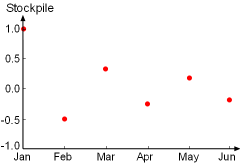Plot of Monthly Stockpile for the
First Six Months

Table: Partial Sum of the
Series (n =12)
 n Sn 1 1 2 0.5 3 0.8333 4 0.5833 5 0.7833 6 0.6167 7 0.7595 8 0.6345 9 0.7456 10 0.6456 11 0.7365 12 0.6532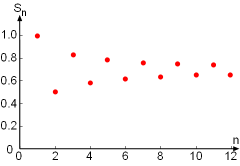The Plot of the Partial Sum Sn
nersus n

Based on the stockpile data on the left, it is noted that the sum of the stockpiles follows the following trend and can be represented by the series: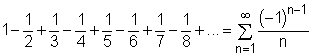where n represents the month (i.e., n = 1 is January, n = 2 is February and so on). It is also recognized that this series is alternating harmonic series.

To determine if the warehouse is suitable or not in long run, the partial sum of the series for n = 12 is obtained as an approximation: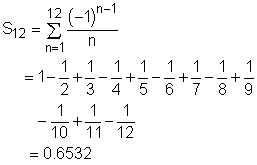Since the warehouse is 65.3% occupied by the end of a year, which is a little more than half, the warehouse is considered suitable for a long period of time.

The error involved in using the partial sum as an approximation for the total sum is given by: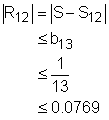The partial sums for the first 12 terms are shown in the figure to show the convergence of the series.